#### Table of Content

25 March 2022, Volume 2022 Issue 2
 For Selected: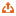Download Citations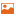Toggle Thumbnails
Mathematics
 Infinite dimensional 3-Pre-Lie algebras Ruipu BAI, Shan LIU 2022, 2022 (2):  1-8.  doi: 10.3969/j.issn.1000-5641.2022.02.001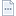Abstract ( 164 )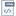HTML ( 292 )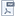PDF (625KB) ( 88 )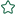Constructing 3-Pre-Lie algebras has always been a difficult problem; until now, there have been very few examples of 3-Pre-Lie algebras. In this paper, we use homogenous Rota-Baxter operators of weight zero on the infinite dimensional 3-Lie algebra $A_{\omega}=\langle L_m | m\in {\mathbb{Z}}\rangle$ to construct 3-Pre-Lie algebras $B_k,~0\leqslant k\leqslant 4$ , and we subsequently discuss the structure. It is shown that $B_2$ and $B_4$ are non-isomorphic simple 3-Pre-Lie algebras, $B_1$ is an indecomposable 3-Pre-Lie algebra with infinitely many one-dimensional ideals, and $B_3$ is an indecomposable 3-Pre-Lie algebra with finitely many ideals.
 The viscosity solution of the discounted Hamilton-Jacobi equation in non-compact space Suting CHEN, Xia LI 2022, 2022 (2):  9-15.  doi: 10.3969/j.issn.1000-5641.2022.02.002Abstract ( 117 )HTML ( 43 )PDF (550KB) ( 53 )The discounted Hamilton-Jacobi equation (H-J equation) is a special form of the contact Hamilton-Jacobi equation; hence, study of the discounted H-J equation is important. In this article, we first study an expression of the viscosity solution $u_{\lambda}(x,t)$ for the discounted H-J equation in non-compact space. Then, we explore the convergence of the viscosity solution $u_{\lambda}(x,t)$ for a specific discounted H-J equation with $\lambda >0$ in non-compact space for the initial value in different cases.
 Vertex-distinguishing IE-total coloring of ${K_{5,\;5,\;p}}$ $(p \geqslant 2\;028)$ Ruimin YAN, Xiang’en CHEN 2022, 2022 (2):  16-23.  doi: 10.3969/j.issn.1000-5641.2022.02.003Abstract ( 90 )HTML ( 41 )PDF (907KB) ( 56 )Let $G$ be a simple graph. A total coloring $f$ of $G$ is called an IE-total coloring if $f(u)\neq f(v)$ for any two adjacent vertices $u$ and $v$ , where $V(G)$ denotes the set of vertices of $G$ . For an IE-total coloring $f$ of $G$ , the set of colors $C(x)$ (non-multiple sets) of vertex $x$ under $f$ of $G$ is the set of colors of vertex $x$ and of the edges incident with $x$ . If any two distinct vertices of $G$ have distinct color sets, then $f$ is called a vertex-distinguishing IE-total coloring of $G$ . We explore the vertex distinguishing IE-total coloring of complete tripartite graphs $K_{5,5,p}$ $(p \geqslant 2\;028)$ through the use of multiple methods, including distributing the color sets in advance, constructing the colorings, and contradiction. The vertex-distinguishing IE-total chromatic number of $K_{5,5,p}$ $(p \geqslant 2\;028)$ is determined.
 Codimension 3 bifurcation of a delayed predator-prey system with double Allee effect Jianfeng JIAO, Can CHEN 2022, 2022 (2):  24-33.  doi: 10.3969/j.issn.1000-5641.2022.02.004Abstract ( 97 )HTML ( 34 )PDF (580KB) ( 48 )By generalizing and using the normal form theory and center manifold theorem of delay differential equations, a class of high-codimension bifurcation problems of predator-prey systems with delay and Allee effect are investigated. Firstly, sufficient conditions for the existence of the positive equilibrium and the codimension 3 bifurcation at this positive equilibrium are established. Subsequently, the normal form of the system at the positive equilibrium is deduced. Finally, from the topological equivalence of the normal form and the original system, the bifurcation phenomenon of the original system at the positive equilibrium is analyzed.
 Determination of convergence control parameters in homotopy analysis solutions based on machine learning technique Tonghui ZHOU, Yinping LIU 2022, 2022 (2):  34-44.  doi: 10.3969/j.issn.1000-5641.2022.02.005Abstract ( 107 )HTML ( 33 )PDF (860KB) ( 55 )Homotopy analysis method is an effective method for constructing approximate analytical solutions to strongly nonlinear problems. The technique has been widely applied to solve important problems in scientific research and engineering technology. Compared with other existing techniques, this method leverages auxiliary parameters and functions to adjust and control the convergence region and convergence speed of approximate analytical solutions. In this paper, we present a parameter selection algorithm based on machine learning techniques to determine the optimal values of convergence control parameters for homotopy analysis solutions. This marks the first time that homotopy analysis method and machine learning techniques have been combined to obtain approximate analytical method with better convergence for strongly nonlinear mathematical and physical equations. By applying the method to several examples, we show that the convergence of solutions using the proposed method is better than those obtained from existing homotopy analysis methods. In addition, our algorithm is both more universal and flexible.
Computer Science
 Coupled propagation dynamics of different time evolution scales on double-layer networks Yingqi ZENG, Min TANG 2022, 2022 (2):  45-54.  doi: 10.3969/j.issn.1000-5641.2022.02.006Abstract ( 97 )HTML ( 38 )PDF (1048KB) ( 38 )Using double-layer networks, we constructed a coupled propagation model (Noisy Voter - Susceptible-Infected-Recovery) with different time evolution scales. This coupled spreading process can be characterized by numerical analysis method of microscopic Markov chain theory. We verified the accuracy of the proposed numerical analysis method using a large number of Monte Carlo simulation experiments. We found a crossover phenomenon of the phase transition type in the coupled model. Specifically, when the noise in the opinion formation process is relatively small, the information propagation scale and the proportion of positive opinions change discontinuously with the information transmission rate. At the same time, the hysteresis loop and bistability phenomenon appear, in which the phenomenon of global consensus can be observed. When the noise is large, the order parameters of these two dynamic processes vary continuously with the transmission rate.
 Recognition of classroom learning behaviors based on the fusion of human pose estimation and object detection Zejie WANG, Chaomin SHEN, Chun ZHAO, Xinmei LIU, Jie CHEN 2022, 2022 (2):  55-66.  doi: 10.3969/j.issn.1000-5641.2022.02.007Abstract ( 182 )HTML ( 43 )PDF (1026KB) ( 229 )As a result of ongoing advances in artificial intelligence technology, the potential for learning analysis in teaching evaluation and educational data mining is gradually being recognized. In classrooms, artificial intelligence technology can help to enable automated student behavior analysis, so that teachers can effectively and intuitively grasp students’ learning behavior engagement; the technology, moreover, can provide data to support subsequent improvements in learning design and implementation of teaching interventions. The main scope of the research is as follows: Construct a classroom student behavior dataset that provides a basis for subsequent research; Propose a behavior detection method and a set of feasible, high-precision behavior recognition models. Based on the global features of the human posture extracted from the Openpose algorithm and the local features of the interactive objects extracted by the YOLO v3 algorithm, student behavior can be identified and analyzed to help improve recognition accuracy; Improve the model structure, compress and optimize the model, and reduce the consumption of computing power and time. Four behaviors closely related to the state of learning engagement: listening, turning sideways, bowing, and raising hands are recognized. The accuracy of the detection and recognition method on the verification set achieves 95.45%. The recognition speed and accuracy of common behaviors, such as playing with mobile phones and writing, are greatly improved compared to the original model.
 Bi-directional long short-term memory and bi-directional gated attention networks for text classification Genmei TONG, Min ZHU 2022, 2022 (2):  67-75.  doi: 10.3969/j.issn.1000-5641.2022.02.008Abstract ( 105 )HTML ( 45 )PDF (667KB) ( 33 )In this paper, we propose the construction of a bi-directional fully connected structure for better extraction of context information. We also propose the construction of a bi-directional attention structure for compressing matrices containing rich text features into a vector. The bi-directional fully connected structure and the gated structure are then combined. This research demonstrates that the proposed combined structure has a net positive effect on text classification accuracy. Finally, by combining these three structures and a bi-direction long short-term memory, we propose a new text classification model. Using this model, we obtained competitive results on seven commonly used text classification datasets and achieved state-of-the-art results on five of them. Experiments showed that the combination of these structures can significantly reduce classification errors.
 Design and implementation of automatic correction for college mathematics assignments Qinmin YANG, Zhisong JIANG 2022, 2022 (2):  76-83.  doi: 10.3969/j.issn.1000-5641.2022.02.009Abstract ( 120 )HTML ( 38 )PDF (821KB) ( 49 )By combining information extraction technology, data matching technology, and one-time manual processing, mathematical subjective questions can be transformed into tree-shaped multiple-choice questions. In this study, an automatic correction system for college mathematics assignments was developed by combining modern information and network technology; the system was subsequently trialed in the teaching of entry-level college mathematics courses. The proposed system solves bottlenecks related to automatic grading of subjective mathematics questions, including multiple-choice questions, fill-in-the-blank questions, judgment questions, quiz questions, calculation questions, and proof questions. The system can correct routine exercises for mathematics courses of primary schools, middle schools, and universities so as to achieve more efficient completion. The idea, furthermore, can be applied to various aspects of mathematics teaching, such as previews before class, classroom exercises, reviews after class, preparations for examinations, online examinations, etc. The electronic data collected in the process of automatic correction can subsequently be used for data analysis, teaching guidance, teaching research, and the construction of educational informatization.
Physics and Electronics
 First-principles calculations investigations of two-dimensional transition metal phosphide MnTn+1(M = V, Cr; T = P, As, and Sb) slices Yaqiong ZHANG, Wenhui XIE 2022, 2022 (2):  84-92.  doi: 10.3969/j.issn.1000-5641.2022.02.010Abstract ( 107 )HTML ( 32 )PDF (1827KB) ( 48 )In this paper, the atomic structure, stability, electronic structure, and magnetism of two-dimensional transition metal phosphide MnTn+1 (M = V, Cr; T = P, As, and Sb) slices were systematically studied using the first-principles calculations based on density functional theory. By calculating the formation energy and phonon spectrum, it was determined that only V4As5, Cr2P3, Cr3P4, Cr4P5, Cr2As3, and Cr3As4 are stable two-dimensional magnetic multilayers. The results show that these stable two-dimensional magnetic materials are antiferromagnetic metals. In addition, the electronic structure and the magnetic coupling mechanism of these materials were further analyzed.
 Optically mediated entanglement between Bose-Einstein condensates Shuai GAO, Matthew PREST, Ebubechukwu O. ILO-OKEKE, Manikandan KONDAPPAN, Juan E. ARISTIZABAL-ZULUAGA, Valentin IVANNIKOV, Tim BYRNES 2022, 2022 (2):  93-105.  doi: 10.3969/j.issn.1000-5641.2022.02.011Abstract ( 162 )HTML ( 39 )PDF (1315KB) ( 70 )This paper explores a method for generating optically mediated entanglement between Bose-Einstein condensates (BECs). Using a quantum nondemolition Hamiltonian with BECs placed in a Mach-Zehnder configuration, it is shown that entangled states can be induced by performing measurement on light. In particular, the effects of the entangled state in the presence of decoherence were analyzed. The behavior of the entangled state was found to be sensitive to the atom-light interaction time. The entangled state is relatively stable when the dimensionless interaction time $\tau \lesssim \frac{1}{\sqrt{N}}$ and relatively fragile when the time is greater.
 Calculation of particle motion trajectories in optical force fields Hailei XIE, Junjie DU 2022, 2022 (2):  106-113.  doi: 10.3969/j.issn.1000-5641.2022.02.012Abstract ( 115 )HTML ( 32 )PDF (893KB) ( 65 )In this paper, the motion trajectory of micro-nanoparticles is calculated based on the Euler-Richardson algorithm after the optical force exerted on the particles is determined using Mie scattering theory. The Euler-Richardson algorithm has better calculation accuracy and faster convergence speed than the Euler algorithm and the Euler-Kromer algorithm, and thus is an appropriate approach to describe the trajectory of particles. Hence, the motion trajectory of a nanoparticle in a periodic conservative optical force field is calculated based on the Euler-Kromer algorithm; the results confirm consistency with the physical analysis, further verifying the effectiveness and stability of the approach. The calculation method shown in this paper provides a high-efficiency approach to study optical trapping, transport, sorting of colloidal particles, and biological macromolecules as well as the cooling of macroscopic particles in optical micro-manipulation.
 Spherically symmetric solutions for a black hole surrounded by plasma Qiang LI, Tao WANG 2022, 2022 (2):  114-119.  doi: 10.3969/j.issn.1000-5641.2022.02.013Abstract ( 69 )HTML ( 25 )PDF (649KB) ( 27 )In this paper, the exterior solution for a spherically symmetric black hole surrounded by plasma is studied in detail. After deriving the fundamental governing equations, the analytic solutions under two approximate conditions, ${g_{tt}}{g_{rr}} = - 1$ and $p = 0$ , are investigated. Comparing the two results with the accurate numerical solution, we find that the former approximation offers superior accuracy. This provides a basis for studying the quasinormal modes of perturbations as well as the shadow and ring when the black hole is surrounded by plasma.
 Study of polarized laser-induced ultrafast spin dynamics on [Pt/Co]3MnIr film Wenqi ZHENG, Yu LIU, Hailin HU, Shitao LOU 2022, 2022 (2):  120-126.  doi: 10.3969/j.issn.1000-5641.2022.02.014Abstract ( 79 )HTML ( 26 )PDF (1952KB) ( 41 )In this study, ultrafast spin dynamics on FM-AFM (ferromagnetism-antiferromagnetism) thin film were explored using pump-probe technology with circularly polarized and linear pump beams. Circularly polarized light generates an effective inducting magnetic field, which is called the inverse Faraday effect. The direction of the transient Kerr peak only depends on the angular momentum of photons. The amplitude of the Kerr peak depends on the thickness of the MnIr film. This may be attributed to the fact that the transient Kerr peak originates from the magnetization of paramagnetic electrons. This study may help further the understanding of spin dynamics in HD-AOS (Helicity-Dependent All Optical Switching).
 Numerical optimization of bichromatic adiabatic cooling Chenyang QIAN, Guangjiong DONG 2022, 2022 (2):  127-134.  doi: 10.3969/j.issn.1000-5641.2022.02.015Abstract ( 76 )HTML ( 25 )PDF (1141KB) ( 20 )In recent years, a new laser cooling method—named bichromatic adiabatic cooling—has been developed; however, the method offers low cooling efficiency. In this paper, numerical optimization of the bichromatic adiabatic cooling process has been performed. It is shown that there is an optimal pulse time for the optical fields to achieve the highest cooling efficiency. Moreover, a comparison of the cooling efficiency with the Gaussian light pulse and square pulse shows that the cooling efficiency is insensitive to the pulse shape. Because this cooling method relies on adiabatic evolution of the light field-atom system, it is shown that it is better to slow the speed of the atomic beam to maintain the adiabatic condition. Finally, the effect of spontaneous emission on bichromatic adiabatic cooling is studied. The results show that use of a long pulse significantly reduces cooling efficiency.
 Limit of multi-parameter phase estimation in an actively correlated Mach-Zehnder interferometer WANG Qiang, ZENG Jie, JIAO Gaofeng, YUAN Chunhua 2022, 2022 (2):  135-142.  doi: 10.3969/j.issn.1000-5641.2022.02.016Abstract ( 89 )HTML ( 25 )PDF (3852KB) ( 28 )In this paper, the phase estimation limits of an active-related Mach-Zehnder interferometer with three port inputs and two different input states was studied using quantum Fisher information and quantum Fisher information matrix theory. In the case of an arbitrary light field input to a single port, the effect of the input field fluctuation on the limit of phase estimation is eliminated by the theory of phase averaging and the quantum Fischer information matrix. In the case of a dual port input coherent state, the effect of the fluctuating light field on the estimation limit cannot be eliminated, and the phase estimation limit depends on the initial phase of the two input coherent states.
 Design of a reconfigurable active filter chip Junwei YU, Shengxi DIAO 2022, 2022 (2):  143-154.  doi: 10.3969/j.issn.1000-5641.2022.02.017Abstract ( 87 )HTML ( 24 )PDF (3268KB) ( 60 )Recently, research on multi-mode, multi-band transceivers has garnered significant interest; in this context, the Software-Define Radio (SDR) system is considered a good candidate. To reduce the negative influence of out-of-band interference on transceiver performance of the SDR system, a high out-of-band rejection IF (intermediate frequency) filter with tunable bandwidth and programable gain is proposed. The proposed filter consists of a biquadratic Gm-C filter, a gain-boosting stage, and a 5th-order elliptic filter. In the proposed filter, the variable gain is achieved using a biquadratic Gm-C filter and a gain-boosting stage, and the tunable bandwidth is achieved using capacitor arrays. In addition, a 5th-order elliptic filter is added to improve out-of-band rejection. The post-layout simulation shows that the bandwidth is tuned over a range of 1 MHz–30 MHz, and the minimum out-of-band rejection at twice the bandwidth reaches 44.56 dB. The gain control range is from –20 dB to 20 dB, and the power consumption and active area for the analog counterpart is 5.1 mW and 1.23 mm2, respectively. The proposed filter is suitable for the analog front-end of multi-mode communication terminals.